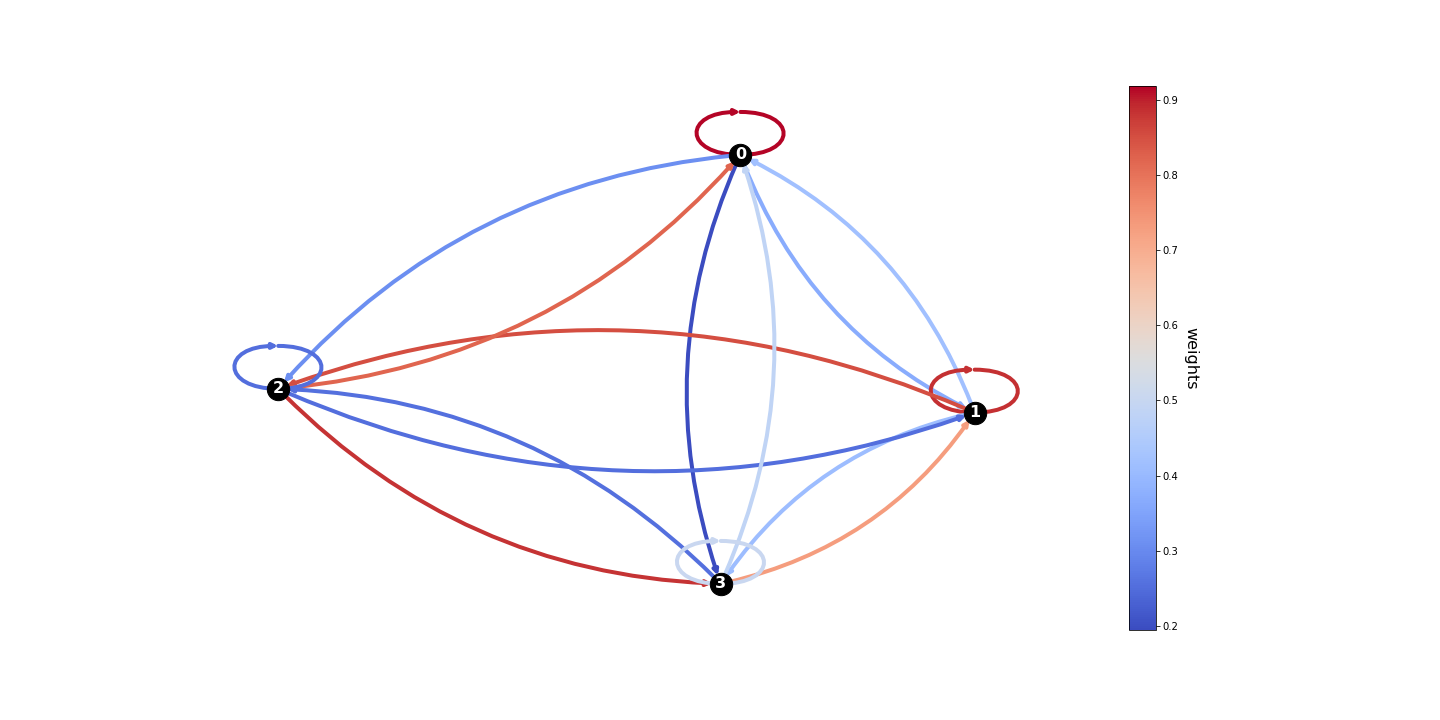# Plotting a weighted graph using networkx

Published:

In this blog post, I show how to plot a weighted graph using networkx.

The following code makes a graph based on a list of nodes and a matrix of edge weights.

For this example, I used the following code the generate the graph and plot the matrix:

>>> n_nodes = 4
>>> M = np.random.rand(n_nodes, n_nodes)
>>> print(M)
[[0.91753808 0.3683666  0.3096244  0.19456242]
[0.41662811 0.88492788 0.85076841 0.40816722]
[0.821422   0.25108361 0.25305956 0.88238645]
[0.48321248 0.73035534 0.25434072 0.50384568]]
>>> nodes = range(M.shape)
>>> G = make_graph(nodes, M)
>>> graphplot(G, M)


The obtained graph is shown below, the weights are encoded as colors of the edges.Tags:

Categories: